# vs.eyeandcontacts.com

## Chapter 8 Motion

Intext Questions
Question 1: An object has moved through a distance. Can it have zero displacement? If yes, support your answer with an example.
Yes, an object can have zero displacement if it has moved through a distance if it comes back to initial position. Example: An athlete runs on a circular track and completes one full round i.e. his initial and final positions are the same.

Question 2: A farmer moves along the boundary of a square field of side 10 m in 40 s. What will be the magnitude of displacement of the farmer at the end of 2 minutes 20 seconds from his initial position?

Given
Side of the square field = 10 m
Perimeter of the field = 4 × s = 4 × 10 = 40 m
Time taken by farmer to cover the boundary = 40 s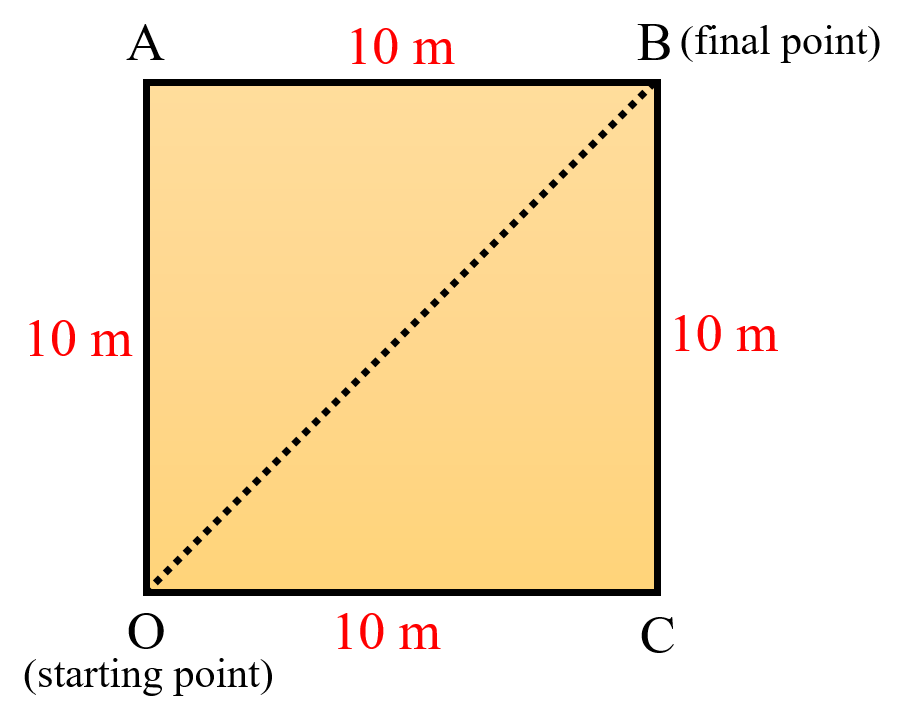We know that, Speed = Distance/Time or v = s/t
Substituting the values, we get
v = 40/40
v = 1 m/s or 1 m s⁻¹

Distance travelled at the end of 2 minutes 20 seconds = Speed × Time or s = v × t

Substituting the values, we get
s = 1([2 × 60] + 20)
s = 1 × 140
s = 140 m

Number of rounds taken by the farmer = Total distance/Total perimeter
= 140/40
= 3.5 rounds

Let us say that the farmer is at B from O after 3.5 rounds.
Displacement at the end of 2 minutes 20 seconds = ?
We know that, a² = b² + c² by Pythagoras theorem
= a² = 10² + 10²
= a² = 200
= a = √200
= a = 14.14 m
Therefore, the displacement of the farmer at the end of 2 minutes 20 seconds will be 14.14 m from his initial position.

Question 3: Which of the following is true for displacement?
a) It cannot be zero.
b) Its magnitude is greater than the distance travelled by the object.
a) False; Displacement can be positive, zero or negative.
b) False; Displacement is always equal to or less than the distance travelled by the object.

Question 4: Distinguish between speed and velocity.
• Speed
→ The distance covered by an object per unit time is called speed.
→ It is a scalar quantity because it has only magnitude.
→ It can be positive only.
• Velocity
→ The distance covered by an object per unit time in a specific direction is called velocity.
→ It is a vector quantity because it has both magnitude and direction.
→ It can be positive, zero or negative.

Question 5: Under what condition(s) is the magnitude of average velocity of an object equal to its average speed?

We know that,
Average speed = Total distance/Total time
Average velocity = Total displacement/Total time
So, the magnitude of average velocity of an object be equal to its average speed when both the total distance and displacement are equal.

Question 6: What does the odometer of an automobile measure?
The odometer of an automobile measures the distance travelled by an automobile.

Question 7: What does the path of an object look like when it is in uniform motion?
The path of an object looks like a straight line when it is in uniform motion.

Question 8: During an experiment, a signal from a spaceship reached the ground station in five minutes. What was the distance of the spaceship from the ground station? The signal travels at the speed of light, that is, 3 × 10⁸ m s⁻¹.

Given
A signal from a spaceship reached the ground station in 5 minutes (300 seconds).
The signal travels at the speed of light, that is, 3 × 10⁸ m s⁻¹.

We know that, Speed = Distance/Time or v = s/t
By rearranging the terms, we get
Distance = Speed × Time or s = v × t
By substituting the values, we get
s = 3 × 10⁸ × 300
s = 900 × 10⁸
s = 9 × 10¹⁰ m
Therefore, the distance of the spaceship from the ground station is 9 × 10¹⁰ m.

Question 9: When will you say a body is in (i) uniform acceleration? (ii) non-uniform acceleration?
i) If an object travels in a straight line and its velocity increases by equal intervals of time, then the acceleration of the object is said to be uniform.
ii) If the changing velocity is not equal in equal intervals of time, then it is said to have non-uniform acceleration or variable acceleration.

Question 10: A bus decreases its speed from 80 km h⁻¹ to 60 km h⁻¹ in 5 s. Find the acceleration of the bus.
Given
Initial speed of the bus = 80 km h⁻¹
Final speed of the bus = 60 km h⁻¹
Time taken to decrease the speed = 5 seconds

We know that, speed with direction is known as velocity.
Since, the magnitude is given in km h⁻¹, it needs to be converted to m s⁻¹.
= 80 × 1000/60 × 60 = 800/36 = 22.22 m s⁻¹
= 60 × 1000/60 × 60 = 100/6 = 16.66 m s⁻¹

We know that, acceleration = final velocity - initial velocity/time or a = v - u/t.
Substituting the values, we get
a = 16.66 - 22.22/5
a = -5.56/5
a = 1.112 m⁻²
Therefore, the acceleration of the bus is -1.112 m s⁻². The negative sign indicates that the bus acceleration is decreasing.

Question 11: A train starting from a railway station and moving with uniform acceleration attains a speed 40 km h⁻¹ in 10 minutes. Find its acceleration.
Given
Train starts from a railway station and moves with uniform acceleration attains speed of 40 km h⁻¹ in 10 minutes (600 seconds).
We know that, speed with direction is called velocity.

Since, the magnitude is given in km h⁻¹, it needs to be converted to m s⁻¹.
= 40 × 1000/60 × 60 = 100/3 = 11.11 m s⁻¹
Since, the train was at before starting the initial velocity will be 0 m s⁻¹.

We know that, acceleration = final velocity - initial velocity/time or a = v - u/t
Substituting the values, we get
a = 11.11 - 0/600
a = 11.11/600
a = 0.0185 m s⁻²
Therefore, the acceleration of the bus after 10 minutes will be 0.0185 m s⁻².

Question 12: What is the nature of the distance-time graphs for uniform and non-uniform motion of an object?
For uniform motion, the distance-time graph is a straight line. For non-uniform motion, the distance-time graph is a curve.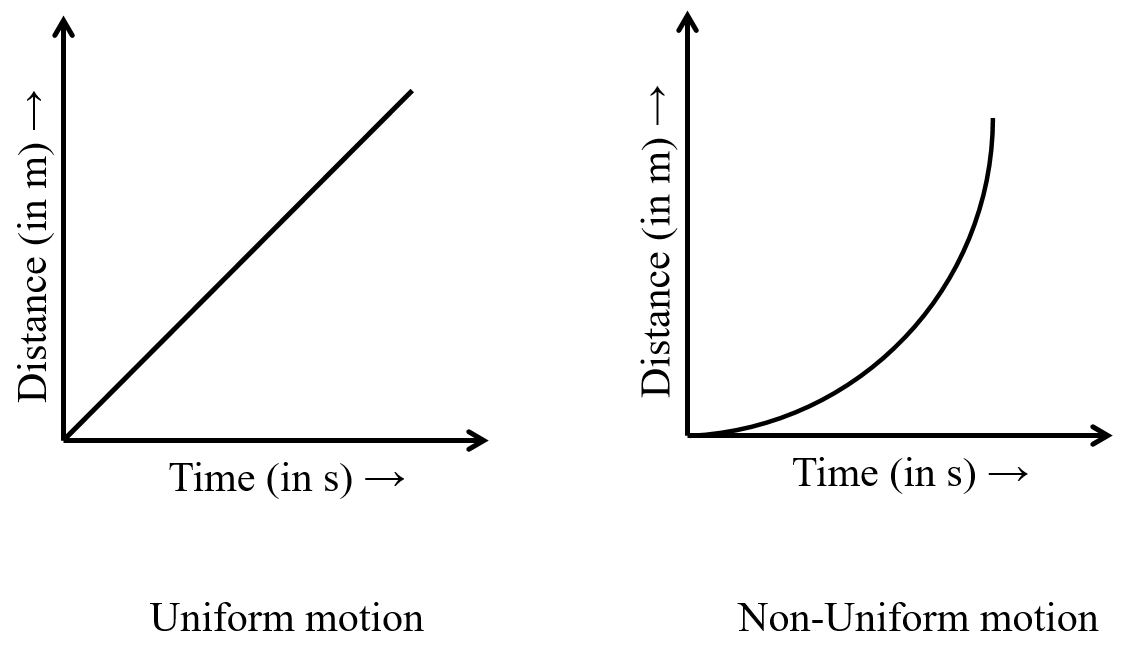Question 13: What can you say about the motion of an object whose distance-time graph is a straight line parallel to the time axis?
When the slope of distance-time graph is parallel to the time axis, the object is at the same position as the time passes that is the object is at rest.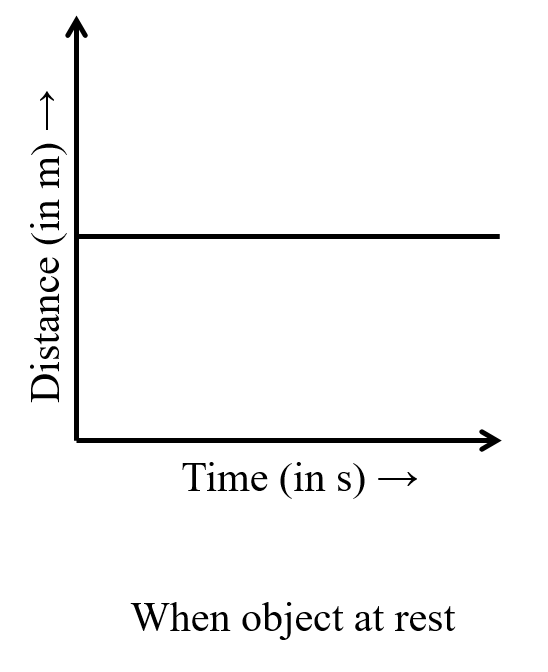Question 14: What can you say about the motion of an object if its speed-time graph is a straight line parallel to the time axis?
If there is no change in the velocity of the object at any interval of time, the object is said to be in uniform motion.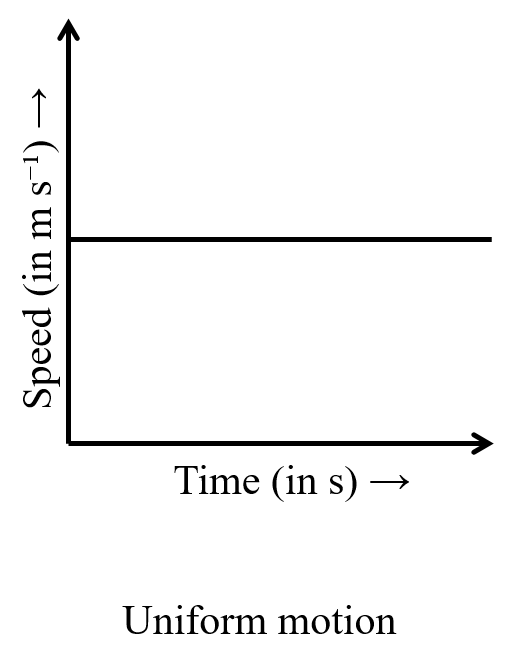Question 15: What is the quantity which is measured by the area occupied below the velocity-time graph?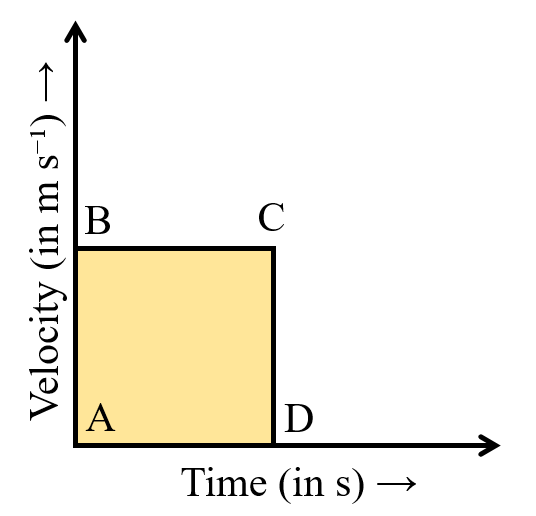Let the velocity of the body move uniformly.
Area of the shaded region = Area of rectangle = l × b
Where l = t and b = v
Area = vt or velocity × time

We know that, velocity = displacement/time or v = s/t
= s/t × t
= s
Therefore, the area occupied by the velocity time graph measures the displacement of the body.

Question 16: A bus starting from rest moves with a uniform acceleration of 0.1 m s⁻² for 2 minutes. Find (a) the speed acquired, (b) the distance travelled.

Given
Initial Velocity of the bus = 0 m s⁻¹
Uniform acceleration of the bus = 0.1 m s⁻²
Time taken to achieve this acceleration = 2 minutes (120 seconds)

a) We know that, acceleration = final velocity - initial velocity/time or a = v - u/t
By rearranging the terms, we get v = u + at
Substituting the values, we get
v = 0 + 0.1 × 120
v = 0 + 12
v = 12 m s⁻¹
Therefore, the speed acquired by the bus in 2 minutes will be 12 m s⁻¹.

b) We know that, s = ut + 1/2at²
Substituting the values, we get
s = 0 × 120 + 1/2 × 0.1 × (120)²
s = 0 + 1/20 + 14400
s = 720 m
Therefore, the distance travelled by the bus in 2 minutes will be 720 m.

Question 17: A train is travelling at a speed of 90 km h⁻¹. Brakes are applied so as to produce a uniform acceleration of -0.5 m s⁻². Find how far the train will go before it is brought to rest.

Given
Initial velocity of the train = 90 km h⁻¹
Uniform acceleration of train = -0.5 m s⁻²
Final velocity of the train = 0 m s⁻¹

Since, the magnitude is given in km h⁻¹, it needs to be converted to m s⁻¹.
= 90 × 1000/60 × 60 = 900/36 = 25 m s⁻¹

We know that, 2as = v² - u²
Substituting the values, we get
2 × -0.5 × s = 0² - 25²
-1 × s = -625
s = -625/-1
s = 625 m
Therefore, the distance travelled by the train before it comes to rest is 625 m.

Question 18: A trolley, while going down an inclined plane, has an acceleration of 2 cm s⁻². What will be its velocity 3 s after the start?

Given
Acceleration of a trolley = 2 cm s⁻² (0.02 m s⁻²)
Initial velocity of the trolley = 0 m s⁻¹
Time = 3 s

We know that, v = u + at
Substituting the values, we get
v = 0 + 0.02 × 3
v = 0.06 m s⁻¹
Therefore, the velocity of the trolley after 3s after start will be 0.06 m s⁻¹.

Question 19: A racing car has a uniform acceleration of 4 m s⁻². What distance will it cover in 10 s after start?

Given
Uniform acceleration of a racing car = 4 m s⁻²
Initial velocity of the racing car = 0 m s⁻¹
Time = 10 s

We know that, s = ut + 1/2at²
Substituting the values, we get
s = 0 × 10 + 1/2 × 4 × 10²
s = 0 + 2 × 100
s = 200 m
Therefore, the racing car will cover 200 m in 10 s after start.

Question 20: A stone is thrown in a vertically upward direction with a velocity of 5 m s⁻¹. If the acceleration of the stone during its motion is 10 m s⁻² in the downward direction, what will be the height attained by the stone and how much time will it take to reach there?

Given
Initial velocity of the stone = 5 m s⁻¹
Acceleration of stone during motion = 10 m s⁻²
Final velocity of the stone = 0 m s⁻¹

We know that, 2as = v² - u²
Substituting the values, we get
2 × 10 × s = 0² - 5²
20 × s = -25
s = -25/20
s = 1.25 m

Time taken to reach there = ?
We know that, v = u + at
Substituting the values, we get
0 = 5 + 10 × t
0 = 5 + 10t
-5 = 10t
t = -5/10
t = 0.5 s
Therefore, the distance attained by the stone is 1.25 m and the time taken to reach there is 0.5 s.

Exercise Questions
Question 1: An athlete completes one round of a circular track of diameter 200 m in 40 s. What will be the distance covered and the displacement at the end of 2 minutes 20 s?

Given
Diameter of the track = 200 m
Circumference of track = 200π metres or 628 m
Distance covered in 1 second = 628/40 or 15.7 m

Distance covered at the end of 2 minutes 20 seconds = 140 × 15.7 = 2198 m
Number of rounds completed by athlete in 140 s = 140/40 = 3.5 rounds

The final position of the athlete (with respect to initial position) is opposite to the initial point, that is the displacement will be the diameter of the track that is 200 m.

Question 2: Joseph jogs from one end A to the other end B of a straight 300 m road in 2 minutes 30 seconds and then turns around and jogs 100 m back to point C in another 1 minute. What are Joseph’s average speeds and velocities in jogging (a) from A to B and (b) from A to C?

Given
Distance between A and B = 300 m
Distance between B and C through A = 400 m
Time taken to travel point A to B = 150 s
Time taken to travel point A to C = 210 s

Displacement from A to B = 300 m
Displacement from A to C = 200 m

We know that, vₐᵥ = Total distance/Total time
Average speed from A to B = 300/150 = 2 m s⁻¹
Average speed from A to C through B = 400/210 = 1.9 m s⁻¹

We know that, vₐᵥ = Total displacement/Total time
Average velocity from A to B = 300/150 = 2 m s⁻¹
Average velocity from A to C through B = 200/210 = 0.95 m s⁻¹

Question 3: Abdul, while driving to school, computes the average speed for his trip to be 20 km h⁻¹. On his return trip along the same route, there is less traffic and the average speed is 30 km h⁻¹. What is the average speed for Abdul’s trip?

Given
Speed from home to school = 20 km h⁻¹
Speed from school to home = 30 km h⁻¹

We know that, vₐᵥ = Total distance/Total time

Let the distance between home and school be x.
Time taken to reach school = t₁
Time taken to reach home = t₂

We know that, time = distance/speed or t = s/v
t₁ = x/20
t₂ = x/30
Substituting the values, we get
= x + x/[x/20 + x/30]
= 2x/[x/12]
= 2x × 12/x
= 2 × 12
= 24 km h⁻¹
Therefore, Abdul’s average speed for entire trip is 24 km h⁻¹.

Question 4: A motorboat starting from rest on a lake accelerates in a straight line at a constant rate of 3.0 m s⁻² for 8.0 s. How far does the boat travel during this time?

Given
Acceleration of motorboat = 3 m s⁻²
Time taken for this acceleration = 8 s
Initial velocity of motorboat = 0 m s⁻¹
Distance travelled in 8 s = ?

We know that, s = ut + 1/2at²
Substituting the values, we get
s = 0 × 8 + 1/2 × 3 × 8²
s = 0 + 32 × 3
s = 96 m
Therefore, the boat travels 96 m in 8 s from rest.

Question 5: A driver of a car travelling at 52 km h⁻¹ applies the brakes and accelerates uniformly in the opposite direction. The car stops in 5 s. Another driver going at 3 km h⁻¹ in another car applies his brakes slowly and stops in 10 s. On the same graph paper, plot the speed versus time graphs for the two cars. Which of the two cars travelled farther after the brakes were applied?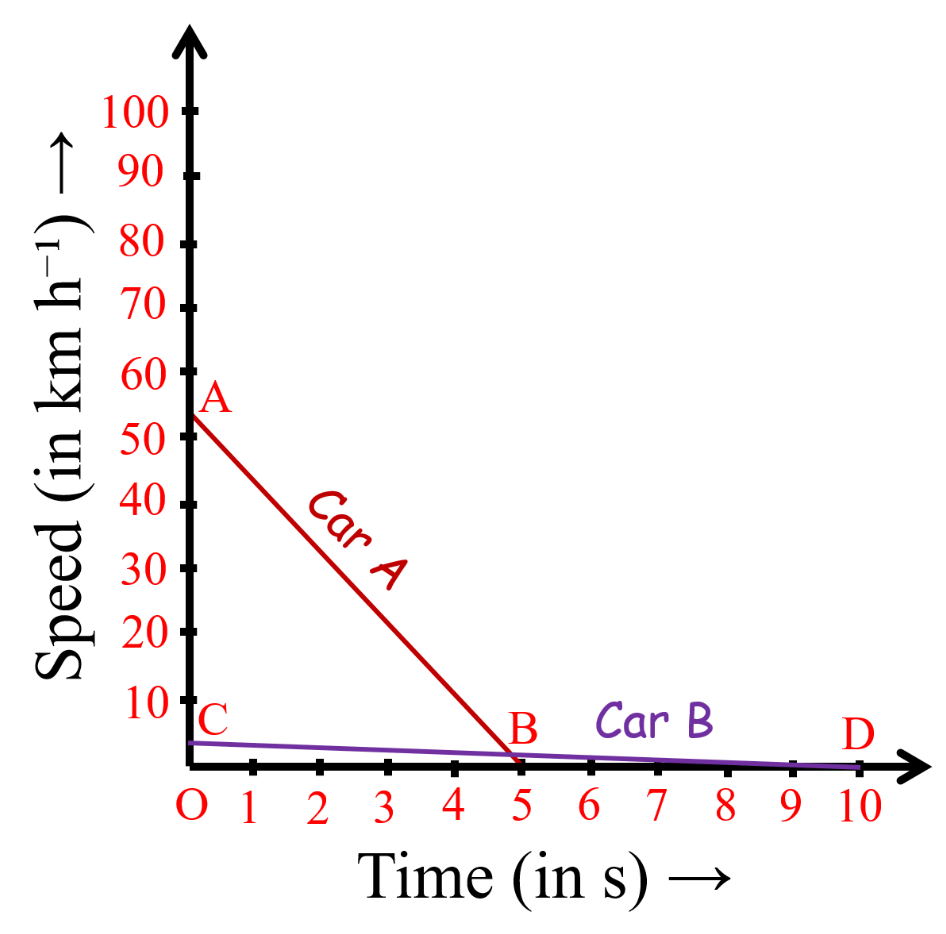Displacement of Car A = area of AOB
= 1/2 × AO × OB
= 1/2 × 5 × 14.44
= 36.1 m

Displacement of Car B = area of COD
= 1/2 × OC × OD
= 1/2 × 0.83 × 10
= 4.15 m
Therefore, the Car A (travelled 52 km h⁻¹) travelled farther by applying brakes.

Question 6: Fig 8.11 shows the distance-time graph of three objects A, B and C. Study the graph and answer the following questions: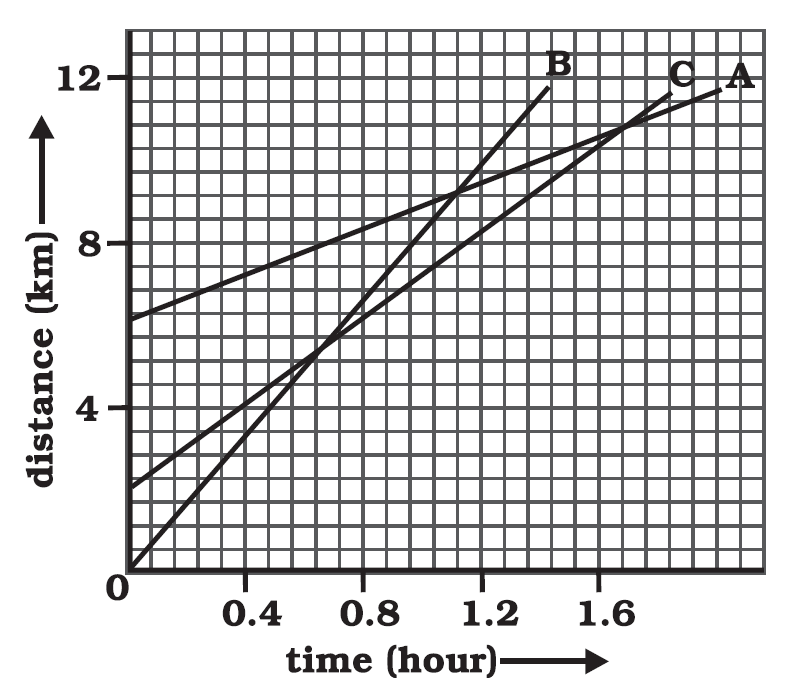a) Which of the three is travelling the fastest?
b) Are all three ever at the same point on the road?
c) How far has C travelled when B passes A?
d) How far has B travelled by the time it passes C?

a) Since, the slope of object B is the greatest, it is travelling the fastest.
b) No, all the three are not at the same point on the road.
c) C has travelled 5.71 km when B passes A.
On the distance axis, 7 small boxes = 4 km. So, 1 small box = 4/7 km.
Initially, C is 4 blocks away from the origin. So, initial distance of C from origin = 16/7
Distance of C from origin when B passes A = 8 km
Distance covered by C
= 8 - 16/7 = 56 - 16/7 = 40/7 = 5.71 km
d) B has travelled 5.14 km when B passes C.
Distance covered by B at the time it passes C = 9 boxes
= 4/7 × 9 = 36/7 = 5.14 km

Question 7: A ball is gently dropped from a height of 20 m. If its velocity increases uniformly at the rate of 10 m s⁻², with what velocity will it strike the ground? After what time will it strike the ground?

Given
Initial velocity of the ball = 0 m s⁻¹
Distance travelled by the ball = 20 m
Acceleration = 10 m s⁻²

We know that, 2as = v² - u²
Substituting the values, we get
2 × 10 × 20 = v² - 0²
400 = v²
v = √400
v = 20 m s⁻¹
Therefore, with 20 m s⁻¹ the ball will strike the ground.

Time it will strike the ground = ?
We know that, a = v - u/t
By rearranging the terms, we get t = v - u/a
Substituting the values, we get
t = 20 - 0/10
t = 20/10
t = 2 s
Therefore, the ball will reach the ground in 2 seconds.

Question 8: The speed-time graph for a car is shown is Fig. 8.12.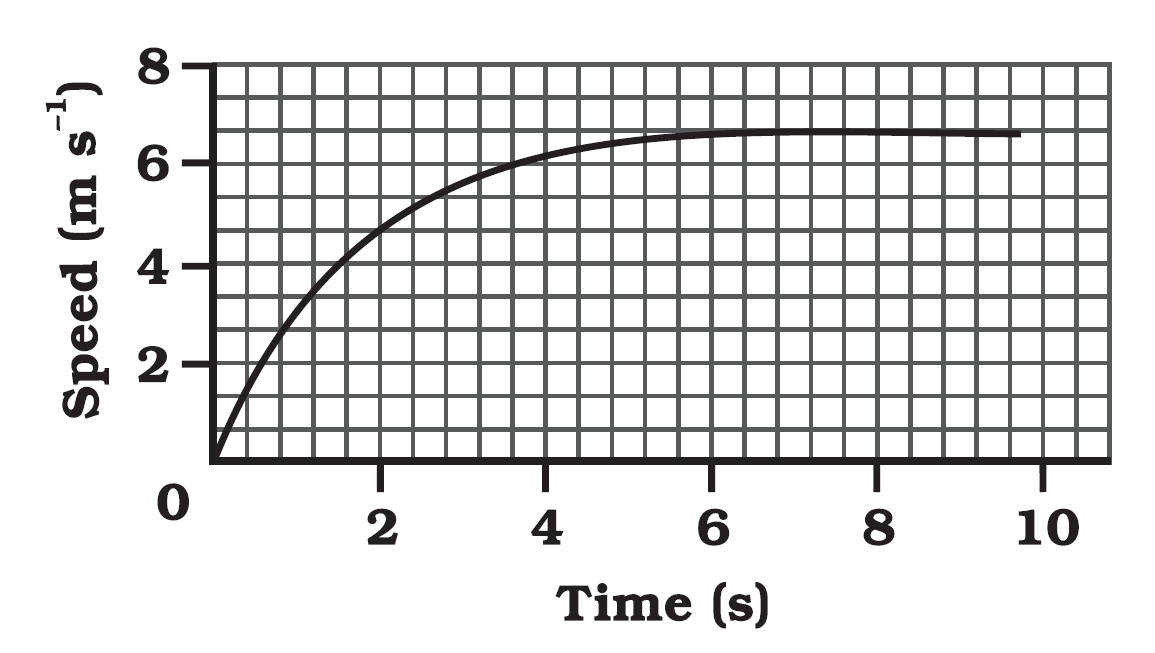a) Find how far does the car travel in the first 4 seconds. Shade the area on the graph that represents the distance travelled by the car during the period.
b) Which part of the graph represents uniform motion of the car?

a) 1/2 × 4 × 6 = 12 m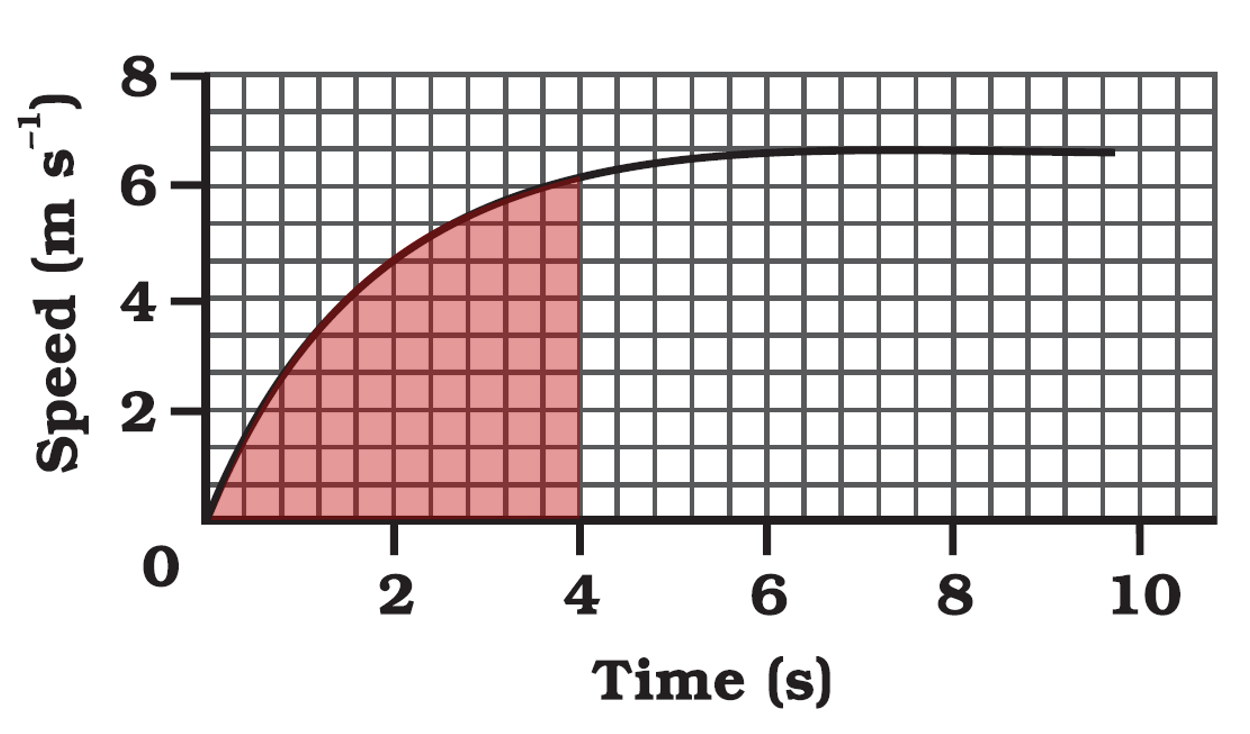b) Since, the speed of the car does not increase from 6th to 10th seconds, the car is said to be in uniform motion.

Question 9: State which of the following situations are possible and give an example for each of these:
a) an object with a constant acceleration but with zero velocity
b) an object moving with an acceleration but with uniform speed
c) an object moving in a certain direction with an acceleration in the perpendicular direction

a) Possible; an object thrown up into the air has a constant acceleration due to gravity acting on it. But, when it reaches its maximum height, its velocity is zero.
b) Possible; when a car is moving in a circular track with constant speed, it is accelerating due to change in direction of motion.
c) Possible; when a car is moving in a circular track, its acceleration is perpendicular to its direction.

Question 10: An artificial satellite is moving in a circular orbit of radius 42250 km. Calculate its speed if it takes 24 hours to revolve around the earth.

Given
Radius of the orbit = 42250 km
Time taken = 24 hr
Speed of satellite = ?

We know that, v = 2πr/t
Substituting the values, we get
v = 2 × 22 × 42250/7 × 24
v = 11 × 21125/7 × 3
v = 11065.47 km h⁻¹
Therefore, the speed of satellite is 11065.47 km h⁻¹.